# Calculate the difference between two dates

Applies to: Excel for Office 365, Excel 2019, Excel 2016, Excel 2013, Excel 2010, Excel 2007.

Use the DATEDIF function for calculating the difference between two dates. Begin by entering a start date in a cell, and an end date in a different cell. Then enter a formula like one of the following.

Warning: If the Start_date is larger than the End_date, the result will return #NUM!.

## Difference in days

In this example, cell D9 contains the start date while the end date is in E9. The formula is found in F9. The “d” calculates the number of full days between the two dates.

## Difference in weeks

In this example, the start date is in cell D13, and the end date is in E13. The “d” yields the number of days. But pay attention to the /7 at the end; this divides the number of days by 7, given a week has 7 days. Note the formatting of this result must be a number. Press CTRL + 1. Then selectNumber > Decimal places: 2.

## Difference in months

In this example, the start date is in cell D5, and the end date is in E5. In the formula, the “m” calculates the number of full months between the two days.

## Difference in years

In this example, the start date is in cell D2, and the end date is in E2. The “y” calculates the amount of full years between the two days.

## Calculate age in accumulated years, months, and days

You can also calculate age or someone’s length of service. The result can be something like “2 years, 4 months, 5 days.”

### 1. Use DATEDIF to find the total years.

In this example, the start date is in cell D17, and the end date is in E17. In the formula, the “y” produces the number of full years between the two days.

### 2. Use DATEDIF again with “ym” to find months.

In another cell, use the DATEDIF formula with the “ym” parameter. The “ym” calculates the number of remaining months past the last full year.

### 3. Use a different formula to find days.

Now we need to find the number of remaining days. We’ll do this by typing a new kind of formula, displayed above. This formula subtracts the first day of the ending month (5/1/2016) from the original end date in cell E17 (5/6/2016).

Here’s how it does this: First the DATE function forms the date, 5/1/2016. It creates it by applying the year in cell E17, and the month in cell E17. Then the 1 symbolises the first day of that month. The result for the DATE function is 5/1/2016. Then, we deduct that from the original end date in cell E17, which is 5/6/2016. 5/6/2016 minus 5/1/2016 is 5 days.

Warning: We don’t suggest using the DATEDIF “md” argument because it could return unreliable results.

### 4. Optional: Combine three formulas in one.

You can integrate all three calculations in one cell like this example. Use ampersands, quotes, and text. It’s a longer formula to enter, but saves time adding extra formulae.

Tip: Press ALT+ENTER to insert line breaks in your formula for easy reading. Also, press CTRL+SHIFT+U if the entire formula isn’t displayed.

## Calculate between today and another date

As already shown to you above, the DATEDIF function calculates the difference between a start date and an end date. Conversely, rather than entering particular dates, you can also utilise the TODAY() function within the formula. After you apply the TODAY() function, Excel captures your computer’s present date for the date. Remember that this will update once the file is opened subsequently on a future day.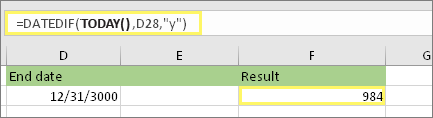Please note that at the time of this writing, the day was October 6, 2016.

## Calculate workdays, with or without holidays

Use the NETWORKDAYS.INTL function anytime you seek to calculate the overall workdays between two dates. Further to this, you can even set it to omit weekends and holidays as well.

Before you begin: Consider if you intend to skip holiday dates. If you do, enter a list of holiday dates in a separate location or sheet. Place every holiday date in its own cell. Then pick those cells, click Formulas > Define Name. Label the range MyHolidays, and press OK. Then make the formula using the steps below.

### 1. Type a start date and an end date.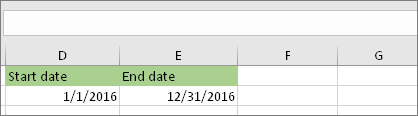In this example, the start date belongs in cell D53 and the end date is found in cell E53.

### 2. In another cell, type a formula like this: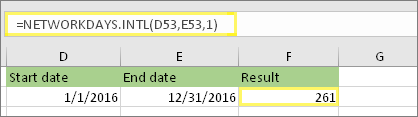Write a formula such as the above example. The 1 in the formula assigns Saturdays and Sundays as weekend days, and removes them from the total.

Note: Excel 2007 doesn’t have the NETWORKDAYS.INTL function. However, it does have NETWORKDAYS. The above example would be like this in Excel 2007: =NETWORKDAYS(D53,E53). You don’t define the 1 because NETWORKDAYS defines the weekend is on Saturday and Sunday.

### 3. If necessary, change the 1.If Saturday and Sunday are not your weekend days, then alter the 1 to another number from the IntelliSense list. For example, 2 establishes Sundays and Mondays as weekend days.

If you are using Excel 2007, ignore this step. Excel 2007’s NETWORKDAYS function always assumes the weekend is on Saturday and Sunday.

### 4. Type the holiday range name.If you created a holiday range name in the “Before you begin” section above, then write it at the end like this. Should you have no holidays, you can skip the comma and MyHolidays out. If you are using Excel 2007, the above example would be this instead: =NETWORKDAYS(D53,E53,MyHolidays).

Tip: If you don’t want to reference a holiday range name, you can also enter a range instead, like D35:E:39. Or, you could type each holiday inside the formula. For example if your holidays were on January 1 and 2 of 2016, you’d type them like this: =NETWORKDAYS.INTL(D53,E53,1,{“1/1/2016″,”1/2/2016”}). In Excel 2007, it would look like this: =NETWORKDAYS(D53,E53,{“1/1/2016″,”1/2/2016”})

## Calculate elapsed time

You can calculate elapsed time by subtracting one time from another. Initially, enter a start time in a cell, and an end time in another. Ensure you write a full time, including the hour, minutes, and a space before the AM or PM. Here’s how:

### 1. Type a start time and end time.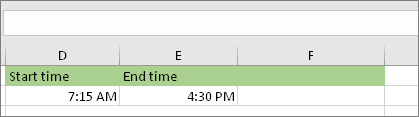In this example, the start time is in cell D80 and the end time is in E80. Check you write the hour, minute, and a space before the AM or PM.

### 2. Set the h:mm AM/PM format.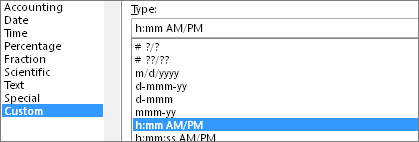Choose both dates and press CTRL + 1 (or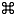+ 1 on the Mac). Ensure to click Custom > h:mm AM/PM, if not previously confirmed.

### 3. Subtract the two times.In another cell, subtract the start time cell from the end time cell.

### 4. Set the h:mm format.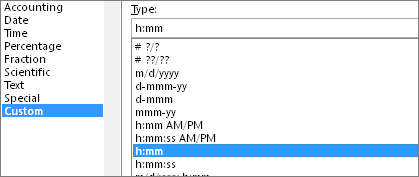Press CTRL + 1 (or+ 1 on the Mac). Pick Custom > h:mm to give the result without AM and PM.

## Calculate elapsed time between two dates and times

To calculate the time between two dates and times, you can merely subtract one from the other. However, you must enforce formatting to every cell to guarantee that Excel generates your sought result.

### 1. Type two full dates and times.In one cell, enter a full start date/time. And in another cell, write a full end date/time. All cells must contain a month, day, year, hour, minute, and a space before the AM or PM.

### 2. Set the 3/14/12 1:30 PM format.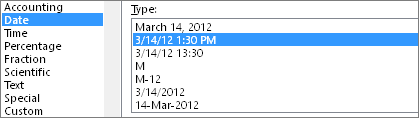Choose both cells, and then press CTRL + 1 (or+ 1 on the Mac). Then pick Date > 3/14/12 1:30 PM. This isn’t the date you’ll set, it’s just a sample of how the format will look. Note that in versions prior to Excel 2016, this format might have a different sample date like 3/14/01 1:30 PM.

### 3. Subtract the two.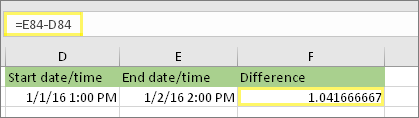In another cell, subtract the start date/time from the end date/time. The result will likely resemble a number and decimal. You’ll fix that in the next step.

### 4. Set the [h]:mm format.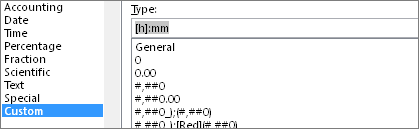Press CTRL + 1 (or+ 1 on the Mac). Choose Custom. In the Type box, enter [h]:mm.